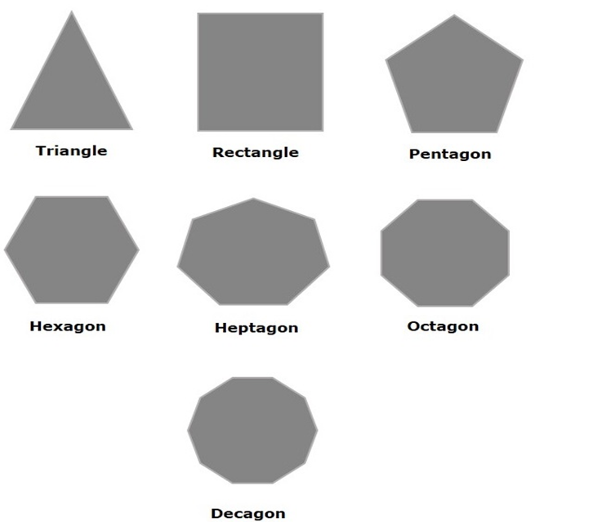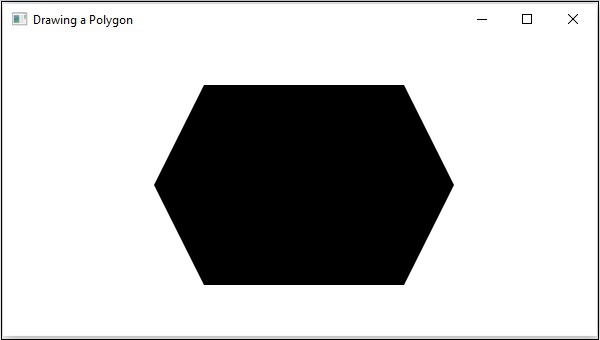# JavaFX - 2D Shapes Polygon

A closed shape formed by a number of coplanar line segments connected end to end.

A polygon is described by two parameters, namely, the length of its sides and the measures of its interior angles.In JavaFX, a polygon is represented by a class named Polygon. This class belongs to the package javafx.scene.shape.

By instantiating this class, you can create a polygon node in JavaFX. You need to pass the x, y coordinates of the points by which the polygon should be defined in the form of a double array.

You can pass the double array as a parameter of the constructor of this class as shown below −

```Polygon polygon = new Polygon(doubleArray);
```

Or, by using the getPoints() method as follows −

```polygon.getPoints().addAll(new Double[]{ List of XY coordinates separated by commas });
```

## Steps to Draw Polygon

To draw a polygon in JavaFX, follow the steps given below.

### Step 1: Creating a Class

Create a Java class and inherit the Application class of the package javafx.application and implement the start() method of this class as follows−

```public class ClassName extends Application {
@Override
public void start(Stage primaryStage) throws Exception {
}
}
```

### Step 2: Creating a Polygon

You can create a polygon in JavaFX by instantiating the class named Polygon which belongs to a package javafx.scene.shape. You can instantiate this class as follows.

```//Creating an object of the class Polygon
Polygon hexagon = new Polygon();
```

### Step 3: Setting Properties to the Polygon

Specify a double array holding the XY coordinates of the points of the required polygon (hexagon in this example) separated by commas, using the getPoints() method of the Polygon class, as follows.

```//Adding coordinates to the hexagon
200.0, 50.0,
400.0, 50.0,
450.0, 150.0,
400.0, 250.0,
200.0, 250.0,
150.0, 150.0,
})
```

### Step 4: Creating a Group Object

In the start() method, create a group object by instantiating the class named Group, which belongs to the package javafx.scene.

Pass the polygon node (hexagon) object, created in the previous step, as a parameter to the constructor of the Group class, in order to add it to the group as follows −

```Group root = new Group(hexagon);
```

### Step 5: Creating a Scene Object

Create a Scene by instantiating the class named Scene which belongs to the package javafx.scene. To this class pass the Group object (root), created in the previous step.

```Scene scene = new Scene(group ,600, 300);
```

### Step 6: Setting the Title of the Stage

You can set the title to the stage using the setTitle() method of the Stage class. The primaryStage is the Stage object which is passed to the start method of the scene class as a parameter.

Using the primaryStage object, set the title of the scene as Sample Application as follows.

```primaryStage.setTitle("Sample Application");
```

### Step 7: Adding Scene to the Stage

You can add a Scene object to the stage using the method setScene() of the class named Stage. Add the Scene object prepared in the previous step using the method as shown below.

```primaryStage.setScene(scene);
```

### Step 8: Displaying the Contents of the Stage

Display the contents of the scene using the method named show() of the Stage class as follows.

```primaryStage.show();
```

### Step 9: Launching the Application

Launch the JavaFX application by calling the static method launch() of the Application class from the main method as follows.

```public static void main(String args[]){
launch(args);
}
```

### Example

Following is a program which generates a Polygon (hexagon) using JavaFX. Save this code in a file with the name PolygonExample.java.

```import javafx.application.Application;
import javafx.scene.Group;
import javafx.scene.Scene;
import javafx.scene.shape.Polygon;
import javafx.stage.Stage;

public class PolygonExample extends Application {
@Override
public void start(Stage stage) {
//Creating a Polygon
Polygon polygon = new Polygon();

300.0, 50.0,
450.0, 150.0,
300.0, 250.0,
150.0, 150.0,
});

//Creating a Group object
Group root = new Group(polygon);

//Creating a scene object
Scene scene = new Scene(root, 600, 300);

//Setting title to the Stage
stage.setTitle("Drawing a Polygon");

stage.setScene(scene);

//Displaying the contents of the stage
stage.show();
}
public static void main(String args[]){
launch(args);
}
}
```

Compile and execute the saved java file from the command prompt using the following commands.

```javac PolygonExample.java
java PolygonExample
```

On executing, the above program generates a JavaFX window displaying a polygon as shown below.javafx_2d_shapes.htm